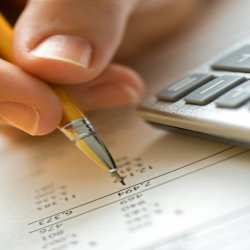Business maths is the  mathematics used by commercial enterprises to record and manage business operations. Commercial organizations use mathematics in accounting, inventory management, marketing, sales forecasting, and financial analysis. Mathematics typically used in commerce includes elementary arithmetic, elementary algebra, statistics and probability. Business management can be made more effective in some cases by use of more advanced mathematics such as calculus, matrix algebra and linear programming.

First let us get an idea of the syllabus:

Unit I : Elementary Financial Mathematics

• A.P., G.P., and sums of their first n terms, Problems with business applications.
• Simple interest, compound interest, interest compounded more than once a year nominal, effective and continuous rates of interest.
• Immediate (ordinary) annuity, its present value and future value.
• Equated Monthly Installments (EMI) using reducing interest system, amortization of loans.
• Sinking Fund.
• Depreciation of assets.
• Functions: Constant function, linear function, step function, xn, exponential and logarithmic functions.
• Business and Economics functions such as demand supply, total revenue, average revenue, total cost, average cost and profit function.
• Equilibrium point.
• Break even analysis.
• Notion of permutations and combinations.

(Problems to be solved with calculator. Use of logarithm tables to be avoided).

Unit II : Matrices and Determinants

• Matrices, Matrix Operations (addition of two matrices, scalar multiple of a matrix, matrix multiplication,      transpose of a matrix)
• Determinant of a matrix of order 2 and 3, elementary properties of determinant, solving a system of linear equations (up to 3 variables) using Cramer’s rule and application to business problems.
• Elementary row and column operations on matrices.
• Inverse of a matrix (up to order 3). Application of matrices to Leontief’s open input-output model. Linear inequality (upto 2 variables ) and their Graphs.

Unit III : Derivatives and their Applications

• Derivatives as a measure of rate. Derivatives of functions constant function xn, ex, ax, log x. Rules of derivatives – scalar multiplication, sum, difference, product and quotient. Second order derivatives. Application of derivatives – marginal cost, marginal revenue, elasticity of demand, maxima and minima for functions in Economics and Business.

Unit IV : Functions of two variables and Integration:

Functions of two variables with examples from Economics and Commerce such as Cobb-Douglas and other production functions, demand functions involving two commodities. First and second order partial derivatives, marginal functions and their simple applications in Economics, Maxima and Minima of functions of two variables using second order partial derivative criterion. Constrained maxima and minima (one constraint only) using Lagrange’s multiplier.

TIPS:

• Make a separate set of notes or book for formulas.
• Revise formulas daily, because confusing them during the exam is a possibility
• Practice a lot because that is the only secret to clearing practical subjects.
• The third unit is the most difficult one with derivatives so make sure you start working on it well in advance.
• The first unit is easy yet lengthy and can store a few tricky questions.
• Unit 2 Matrices and Unit 4 Functions are very easy and won’t take much time in understanding or scoring.
• Keep these very easy mantras in mind and scoring in this subject will become the easiest task.
The following two tabs change content below.#### Ami Pandya

'Ever Learning, Ever Evolving, Never Giving up' sums up www.bms.co.in's Content Manager and passionate writer, Ami. She is a BMS graduate who has freelanced in the past with the top Indian newspapers and magazines. Apart from writing she also likes to indulge into travelling, photography and social work.BMS.co.in is aimed at revolutionising Bachelors in Management Studies education, also known as BMS for students appearing for BMS exams across all states of India. We provide free study material, 100s of tutorials with worked examples, past papers, tips, tricks for BMS exams, we are creating a digital learning library.

Disclaimer: We are not affiliated with any university or government body in anyway.

©2020 BMS - Bachelor of Management Studies Community